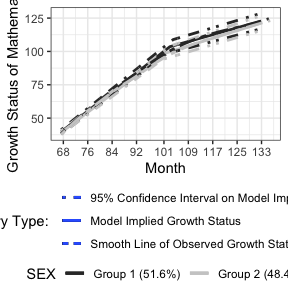# Multiple-group Longitudinal Models

## Load nlpsem package, dependent packages and set CSOLNP as the optimizer

library(nlpsem)
mxOption(model = NULL, key = "Default optimizer", "CSOLNP", reset = FALSE)

load(system.file("extdata", "getMGroup_examples.RData", package = "nlpsem"))

## Load example data and preprocess data

# Load ECLS-K (2011) data
data("RMS_dat")
RMS_dat0 <- RMS_dat
# Re-baseline the data so that the estimated initial status is for the
# starting point of the study
baseT <- RMS_dat0$T1 RMS_dat0$T1 <- RMS_dat0$T1 - baseT RMS_dat0$T2 <- RMS_dat0$T2 - baseT RMS_dat0$T3 <- RMS_dat0$T3 - baseT RMS_dat0$T4 <- RMS_dat0$T4 - baseT RMS_dat0$T5 <- RMS_dat0$T5 - baseT RMS_dat0$T6 <- RMS_dat0$T6 - baseT RMS_dat0$T7 <- RMS_dat0$T7 - baseT RMS_dat0$T8 <- RMS_dat0$T8 - baseT RMS_dat0$T9 <- RMS_dat0\$T9 - baseT
xstarts <- mean(baseT)

## Example: Fit multiple group bilinear spline LGCM to evaluate the difference in the development of mathematics ability

MGroup_Math_BLS_LGCM_f <-  getMGroup(
dat = RMS_dat0, grp_var = "SEX", sub_Model = "LGCM", y_var = "M", t_var = "T",
records = 1:9, curveFun = "BLS", intrinsic = TRUE, res_scale = list(0.1, 0.1)
)
Figure1 <- getFigure(
model = MGroup_Math_BLS_LGCM_f@mxOutput, nClass = 2, cluster_TIC = NULL, grp_var = "SEX",
sub_Model = "LGCM", y_var = "M", curveFun = "BLS", y_model = "LGCM", t_var = "T",
records = 1:9, m_var = NULL, x_var = NULL, x_type = NULL, xstarts = xstarts,
xlab = "Month", outcome = "Mathematics"
)
#> Treating first argument as an object that stores a character
#> Treating first argument as an object that stores a character
show(Figure1)
#> figOutput Object
#> --------------------
#> Trajectories: 1
#> Figure 1:
#> geom_smooth() using method = 'gam' and formula = 'y ~ s(x, bs = "cs")'